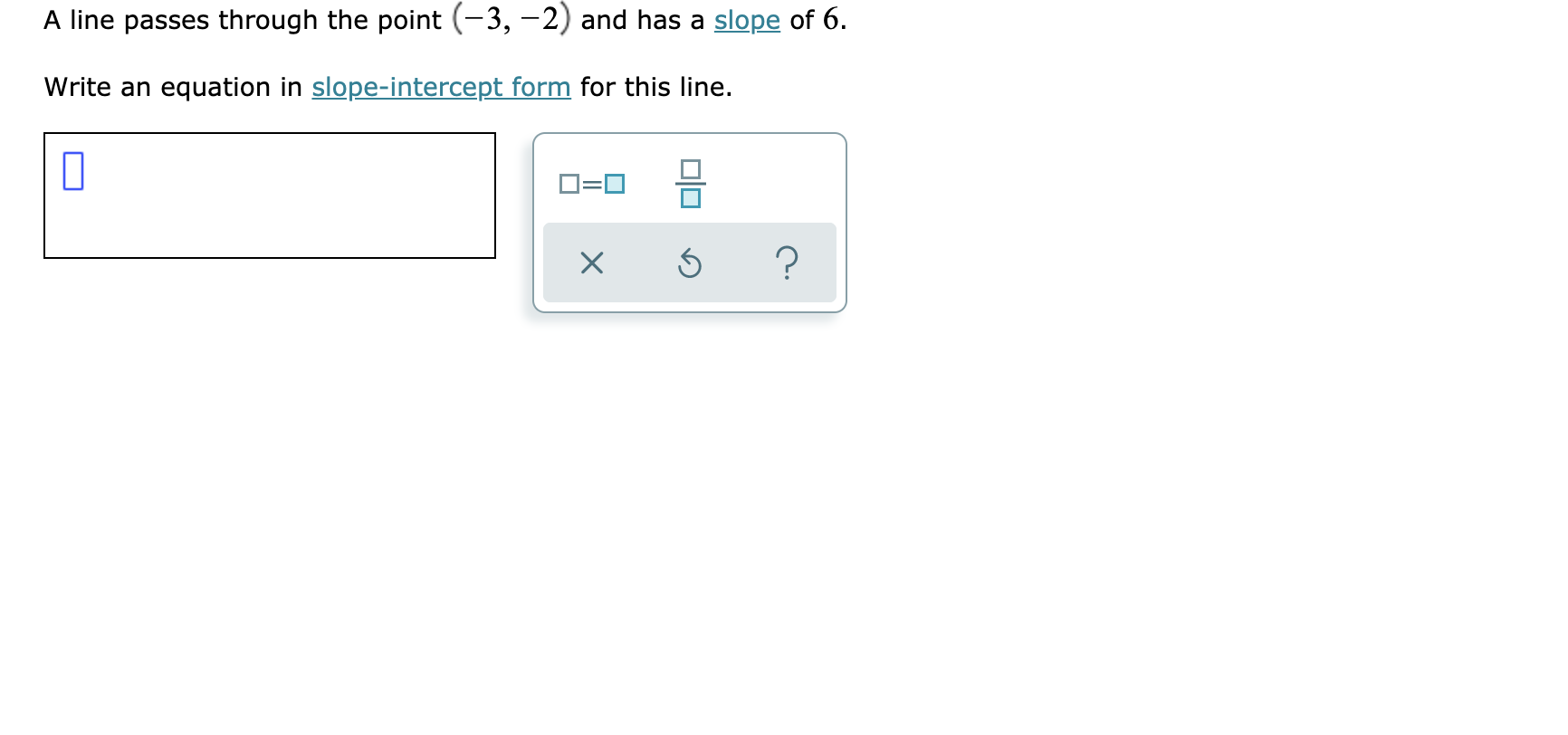# A line passes through the point (-3, -2) and has a slope of 6Write an equation in slope-intercept form for this line.O=0?

Question
3 views

See attachedhelp_outlineImage TranscriptioncloseA line passes through the point (-3, -2) and has a slope of 6 Write an equation in slope-intercept form for this line. O=0 ? fullscreen
check_circle

Step 1

Equation of line in slope intercept form is

Step 2

Given slope of line is m= 6 so putting value of m in equation (1)

Step 3

Given line is passing through point (-3, -2) so th...

### Want to see the full answer?

See Solution

#### Want to see this answer and more?

Solutions are written by subject experts who are available 24/7. Questions are typically answered within 1 hour.*

See Solution
*Response times may vary by subject and question.
Tagged in
MathCalculus

### Other